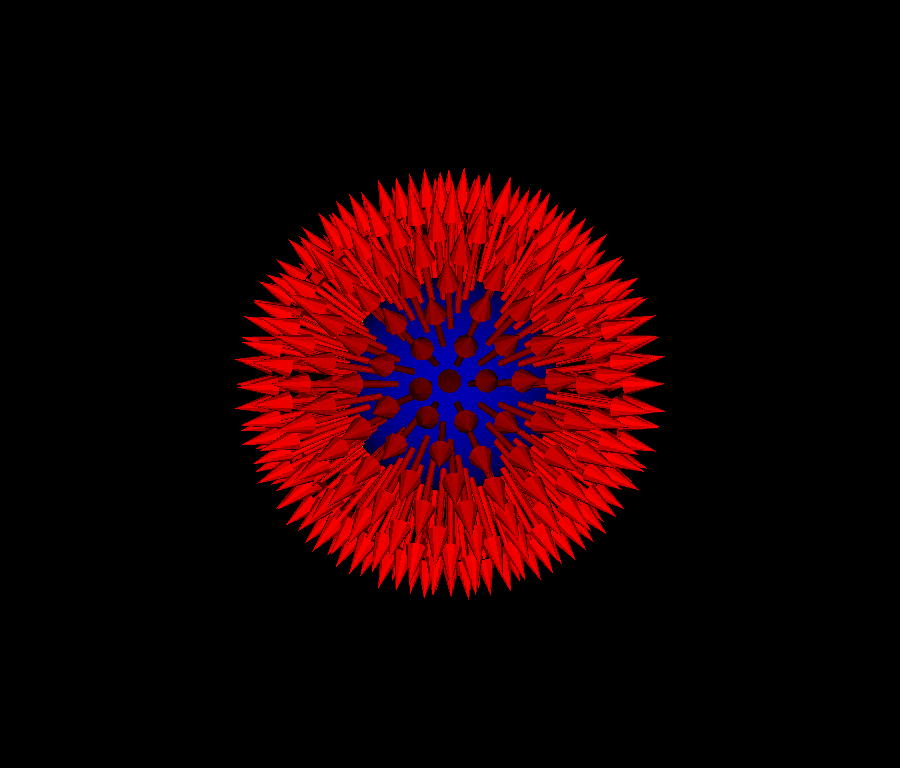# Spiky Sphere#

In this tutorial, we show how to create a sphere with spikes.

```import itertools

import numpy as np

from fury import actor, primitive, utils, window
```

Create a sphere actor. Define the center, radius and color of a sphere. The sphere actor is made of points (vertices) evenly distributed on a sphere. Let’s create a scene.

```scene = window.Scene()
```

The vertices are connected with triangles in order to specify the direction of the surface normal. `prim_sphere` provides a sphere with evenly distributed points

```vertices, triangles = primitive.prim_sphere(name='symmetric362', gen_faces=False)
```

To be able to visualize the vertices, let’s define a point actor with green color.

```point_actor = actor.point(vertices, point_radius=0.01, colors=(0, 1, 0))
```

Normals are the vectors that are perpendicular to the surface at each vertex. We specify the normals at the vertices to tell the system whether triangles represent curved surfaces.

```normals = utils.normals_from_v_f(vertices, triangles)
```

The normals are usually used to calculate how the light will bounce on the surface of an object. However, here we will use them to direct the spikes (represented with arrows). So, let’s create an arrow actor at the center of each vertex.

```arrow_actor = actor.arrow(
centers=vertices,
directions=normals,
colors=(1, 0, 0),
heights=0.2,
resolution=10,
vertices=None,
faces=None,
)
```

To be able to visualize the surface of the primitive sphere, we use `get_actor_from_primitive`.

```primitive_colors = np.zeros(vertices.shape)
primitive_colors[:, 2] = 180
primitive_actor = utils.get_actor_from_primitive(
vertices=vertices,
triangles=triangles,
colors=primitive_colors,
normals=normals,
backface_culling=True,
)
```

We add all actors (visual objects) defined above to the scene.

```scene.add(point_actor)
```

The ShowManager class is the interface between the scene, the window and the interactor.

```showm = window.ShowManager(
scene, size=(900, 768), reset_camera=False, order_transparent=True
)
```

We want to make a small animation for fun! We can determine the duration of animation with using the `counter`. Use itertools to avoid global variables.

```counter = itertools.count()
```

The timer will call this user defined callback every 200 milliseconds. The application will exit after the callback has been called 20 times.

```def timer_callback(_obj, _event):
cnt = next(counter)
showm.scene.azimuth(0.05 * cnt)
primitive_actor.GetProperty().SetOpacity(cnt / 10.0)
showm.render()
if cnt == 20:
showm.exit()